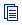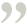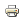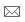Author Informationmahan has 352 Published Articles India, rajasthan, jaipur, jaipur, jaipur

### algebra tutor and algebra equation

Posted On : Sep-30-2011 | seen (348) times | Article Word Count : 485 |Algebra is an important branch of mathematics that concerns the study of the rules of operations and relations. It includes various conceptsAlgebra is an important branch of mathematics that concerns the study of the rules of operations and relations. It includes various concepts that arise from the rules of operations. Other important branches of mathematics other than algebra are geometry, trigonometry, number theory, analysis, topology, combination and many others. Algebra is roughly divided into few categories like elementary algebra, abstract algebra, linear algebra, universal algebra, algebraic number theory algebraic geometry and algebraic combination. I will give a brief idea about all these different types of algebra. Elementary algebra is one in which the properties of operations are noted using different symbols like place holders to denote constants and variables on the real number system. This is generally taught at school level. Abstract algebra is also called as modern algebra, in this, we study about the groups, rings and fields. Study of matrices or vector space properties is called as linear algebra. In universal algebra, we study the common properties of all algebraic structures. Algebraic number theory is the study of properties of numbers through algebraic systems. In algebraic geometry, we apply the abstract algebraic concept to the problems of geometry.

To solve any algebraic question, we need an algebra equations. If the question is directly given in the form of equations then no need to worry but if the question is in the form of word problem or some other type then first form the equation by evaluating the data given. After that you will be able to solve the question. For solving the algebra equations there are two commonly used methods elimination and substitution method. I will explain you how to solve algebra equations.. Let’s take an example to see how to solve algebraic equations.

x+ y = 2

2x – y = 4

Step 1: add both the equations, on adding we get

x+ y +2x –y = 2+4 ,

3x = 6

Step 2: take 3 in denominator of 6 and we get x = 2

x = 6/3 = 2 .

Step 3: now, put value of x in any of the equation lets put in first one we get

2 + y = 2,

y= 2-2

y = 0.

Thus we calculated both the values using elimination method.

If you want to learn algebra equations in deep then you can take advantage of algebra help online. In this article I can tell you about algebra problems but you can’t put your queries in front of me. So solve your queries with the help of algebra tutors. They are available all the time and you can discuss any algebraic topic with them. We have one equation and variable then we take solve it by doing simple calculations but if we have two equations and two variables then we use the above methods.

Author Resource :

TutorVista is the #1 portal for learning algebra tutor online. The tutors working with us are great in explaining algebra help online in best possible way.

Keywords :Print this ArticleSend to Friend